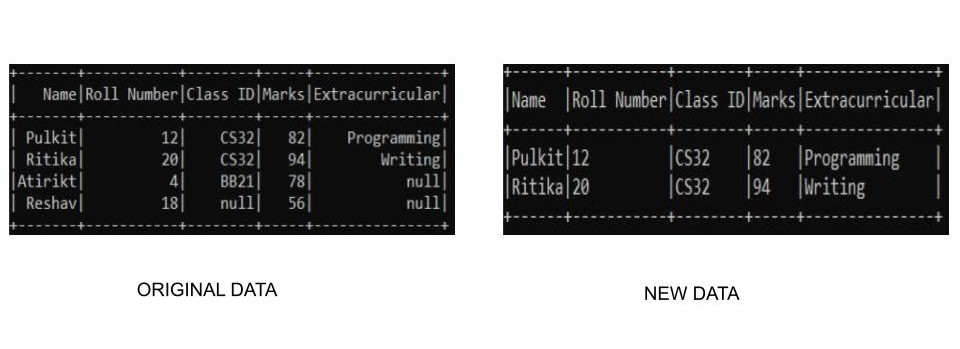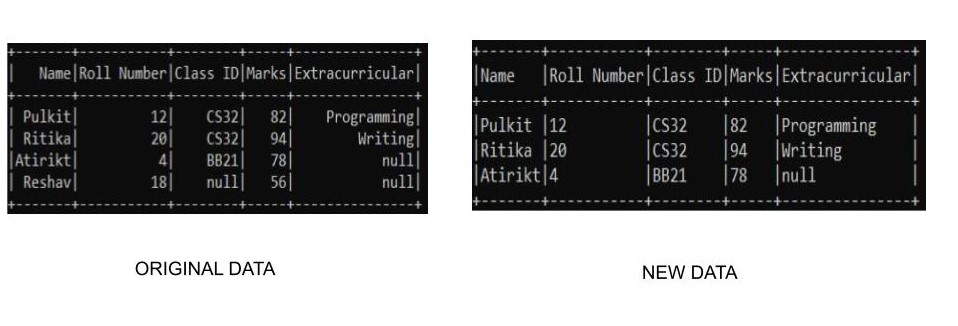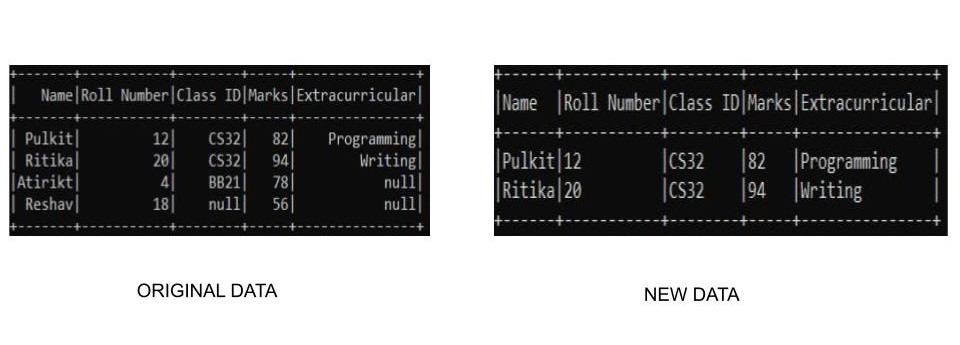# PySpark DataFrame – Drop Rows with NULL or None Values

• Last Updated : 30 Apr, 2021

Sometimes while handling data inside a dataframe we may get null values. In order to clean the dataset we have to remove all the null values in the dataframe. So in this article, we will learn how to drop rows with NULL or None Values in PySpark DataFrame.

### Function Used

In pyspark the drop() function can be used to remove null values from the dataframe. It takes the following parameters:-

Syntax: dataframe_name.na.drop(how=”any/all”,thresh=threshold_value,subset=[“column_name_1″,”column_name_2”])

• how – This takes either of the two values ‘any’ or ‘all’.  ‘any’, drop a row if it contains NULLs on any columns and ‘all’, drop a row only if all columns have NULL values. By default it is set to ‘any’
• thresh – This takes an integer value and drops rows that have less than that thresh hold non-null values. By default it is set to ‘None’.
• subset – This parameter is used to select a specific column to target the NULL values in it. By default it’s ‘None

Note: DataFrame has a variable na which represents an instance of class DataFrameNaFunctions. Thus, we use na variable on DataFrame to use drop() function.

We are specifying our path to spark directory using the findspark.init() function in order to enable our program to find the location of apache spark in our local machine. Ignore this line if you are running the program on cloud. Suppose we have our spark folder in c drive by name of spark so the function would look something like :- findspark.init(‘c:/spark’). Not specifying the path sometimes may lead to py4j.protocol.Py4JError error when running the program locally.

### Examples

Example 1: Dropping All rows with any Null Values

In this example, we are going to create our own custom dataset and use the drop() function to eliminate the rows that have null values. We are going to drop all the rows in that have Null values in the dataframe. Since we are creating our own data we need to specify our schema along with it in order to create the dataset.

## Python3

 `from` `pyspark.sql.types ``import` `StructType, StructField, StringType, IntegerType, FloatType ` `from` `pyspark.sql ``import` `SparkSession ` `import` `findspark ` ` `  ` `  `# spark location ` `# add the respective path to your spark ` `findspark.init(``'_path-to-spark_'``) ` ` `  `# Initialize our data ` `data2 ``=` `[(``"Pulkit"``, ``12``, ``"CS32"``, ``82``, ``"Programming"``), ` `         ``(``"Ritika"``, ``20``, ``"CS32"``, ``94``, ``"Writing"``), ` `         ``(``"Atirikt"``, ``4``, ``"BB21"``, ``78``, ``None``), ` `         ``(``"Reshav"``, ``18``, ``None``, ``56``, ``None``) ` `         ``] ` ` `  `# Start spark session ` `spark ``=` `SparkSession.builder.appName(``"Student_Info"``).getOrCreate() ` ` `  `# Define schema ` `schema ``=` `StructType([ ` `    ``StructField(``"Name"``, StringType(), ``True``), ` `    ``StructField(``"Roll Number"``, IntegerType(), ``True``), ` `    ``StructField(``"Class ID"``, StringType(), ``True``), ` `    ``StructField(``"Marks"``, IntegerType(), ``True``), ` `    ``StructField(``"Extracurricular"``, StringType(), ``True``) ` `]) ` ` `  `# create the dataframe ` `df ``=` `spark.createDataFrame(data``=``data2, schema``=``schema) ` ` `  `# drop None Values ` `df.na.drop(how``=``"any"``).show(truncate``=``False``) ` ` `  `# stop spark session ` `spark.stop() `

Output:Example 2: Dropping All rows with any Null Values in Specific Column

We can also select particular columns to check from by using the subset field. In this example we are using our custom-built dataset and will remove the data of the row which has null value in Class ID column only.  Since we are creating our own data we need to specify our schema along with it in order to create the dataset. We can perform the operation in the following way:-

## Python3

 `from` `pyspark.sql.types ``import` `StructType, StructField, StringType, IntegerType, FloatType ` `from` `pyspark.sql ``import` `SparkSession ` `import` `findspark ` ` `  ` `  `# spark location ` `# add the respective path to your spark ` `findspark.init(``'_path-to-spark_'``) ` ` `  `# Initialize our data ` `data2 ``=` `[(``"Pulkit"``, ``12``, ``"CS32"``, ``82``, ``"Programming"``), ` `         ``(``"Ritika"``, ``20``, ``"CS32"``, ``94``, ``"Writing"``), ` `         ``(``"Atirikt"``, ``4``, ``"BB21"``, ``78``, ``None``), ` `         ``(``"Reshav"``, ``18``, ``None``, ``56``, ``None``) ` `         ``] ` ` `  `# Start spark session ` `spark ``=` `SparkSession.builder.appName(``"Student_Info"``).getOrCreate() ` ` `  `# Define schema ` `schema ``=` `StructType([ ` `    ``StructField(``"Name"``, StringType(), ``True``), ` `    ``StructField(``"Roll Number"``, IntegerType(), ``True``), ` `    ``StructField(``"Class ID"``, StringType(), ``True``), ` `    ``StructField(``"Marks"``, IntegerType(), ``True``), ` `    ``StructField(``"Extracurricular"``, StringType(), ``True``) ` `]) ` ` `  `# create the dataframe ` `df ``=` `spark.createDataFrame(data``=``data2, schema``=``schema) ` ` `  `# drop None Values ` `df.na.drop(subset``=``[``"Class ID"``]).show(truncate``=``False``) ` ` `  `# stop spark session ` `spark.stop() `

Output:Example 3: Dropping All rows with any Null Values Using dropna() method

A third way to drop null valued rows is to use dropna() function. The dropna() function performs in the similar way as of na.drop() does. Here we don’t need to specify any variable as it detects the null values and deletes the rows on it’s own. Since we are creating our own data we need to specify our schema along with it in order to create the dataset. We can use it in pyspark in the following way:-

## Python3

 `from` `pyspark.sql.types ``import` `StructType, StructField, StringType, IntegerType, FloatType ` `from` `pyspark.sql ``import` `SparkSession ` `import` `findspark ` ` `  ` `  `# spark location ` `# add the respective path to your spark ` `findspark.init(``'_path-to-spark_'``) ` ` `  `# Initialize our data ` `data2 ``=` `[(``"Pulkit"``, ``12``, ``"CS32"``, ``82``, ``"Programming"``), ` `         ``(``"Ritika"``, ``20``, ``"CS32"``, ``94``, ``"Writing"``), ` `         ``(``"Atirikt"``, ``4``, ``"BB21"``, ``78``, ``None``), ` `         ``(``"Reshav"``, ``18``, ``None``, ``56``, ``None``) ` `         ``] ` ` `  `# Start spark session ` `spark ``=` `SparkSession.builder.appName(``"Student_Info"``).getOrCreate() ` ` `  `# Define schema ` `schema ``=` `StructType([ ` `    ``StructField(``"Name"``, StringType(), ``True``), ` `    ``StructField(``"Roll Number"``, IntegerType(), ``True``), ` `    ``StructField(``"Class ID"``, StringType(), ``True``), ` `    ``StructField(``"Marks"``, IntegerType(), ``True``), ` `    ``StructField(``"Extracurricular"``, StringType(), ``True``) ` `]) ` ` `  `# create the dataframe ` `df ``=` `spark.createDataFrame(data``=``data2, schema``=``schema) ` ` `  `# drop None Values ` `df.dropna().show(truncate``=``False``) ` ` `  `# stop spark session ` `spark.stop() `

Output:My Personal Notes arrow_drop_up
Recommended Articles
Page :